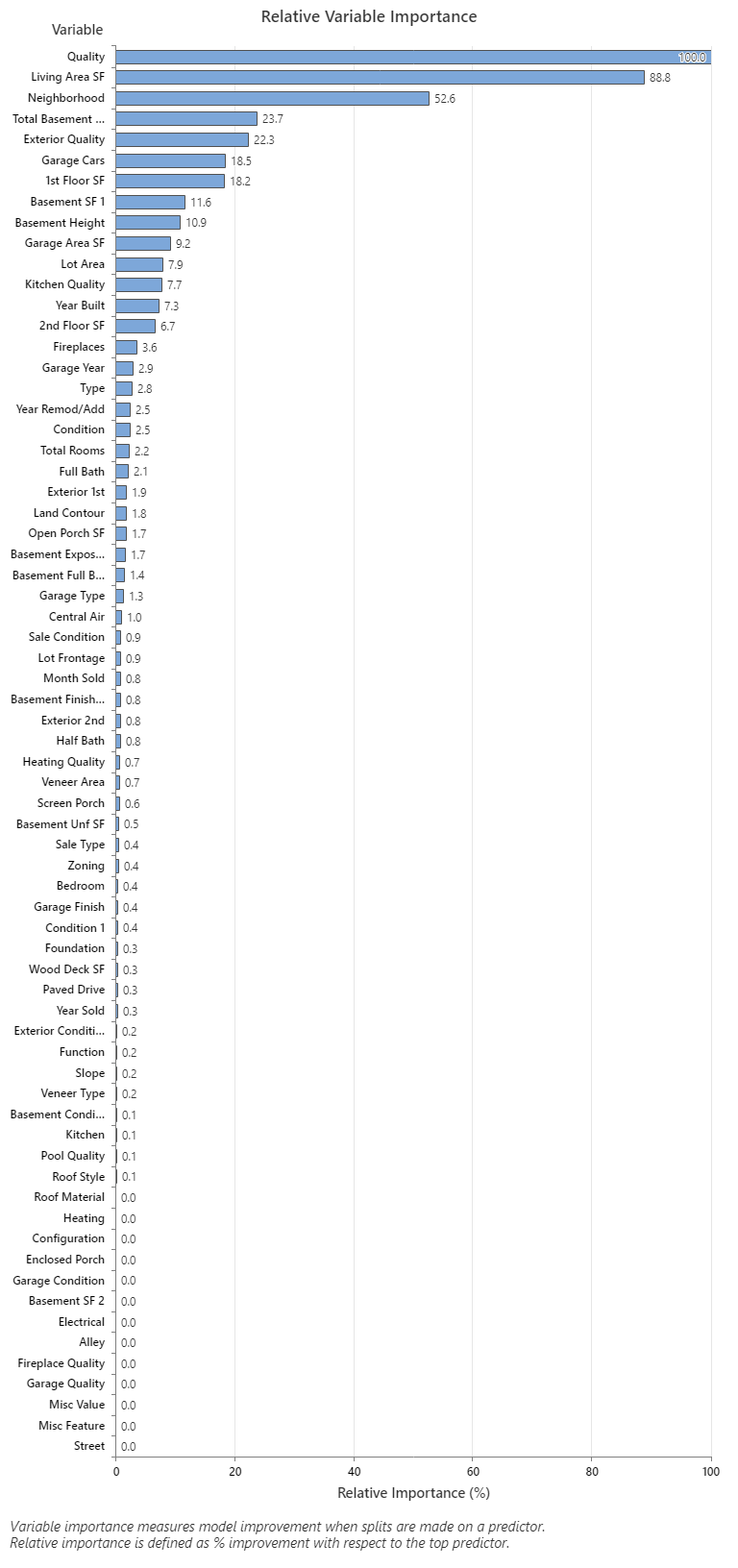# Relative variable importance chart for Random Forests® Regression

###### Note

This command is available with the Predictive Analytics Module. Click here for more information about how to activate the module.

The Relative variable importance graph plots the predictors in order of their effect on model improvement when splits are made on a predictor over the entire forest. The variable with the highest improvement score is set as the most important variable, and the other variables follow in order of importance. Relative variable importance standardizes the importance values for ease of interpretation. Relative importance is defined as the percent improvement with respect to the most important predictor, which has an importance of 100%.

Relative importance is calculated by dividing each variable importance score by the largest importance score of the variables, then we multiply by 100%.

## Interpretation

Relative variable importance values range from 0% to 100%. The most important variable always has a relative importance of 100%. If a variable is not used in the model at all, it is not important.The most important predictor variable for predicting the sale price is Quality. If the importance of the top predictor variable, Quality, is 100%, then the next important variable, Living Area SF, has a contribution of 88.8%. This means that the square footage of the living is 88.8% as important as the overall quality of the property. The next most important variable is Neighborhood which has a contribution of 52.6%.
By using this site you agree to the use of cookies for analytics and personalized content.  Read our policy